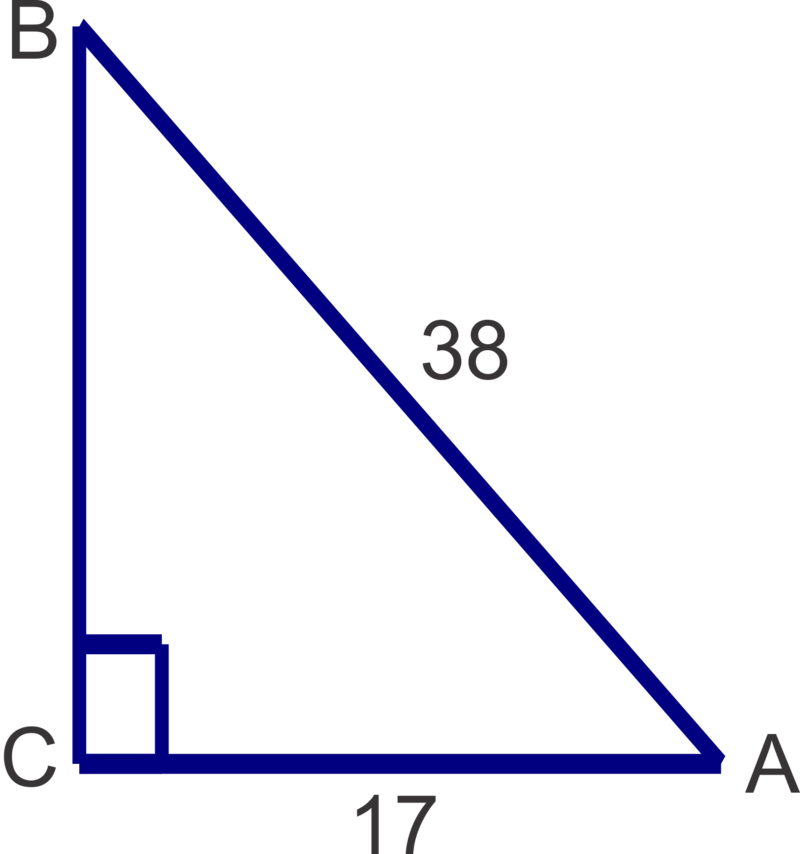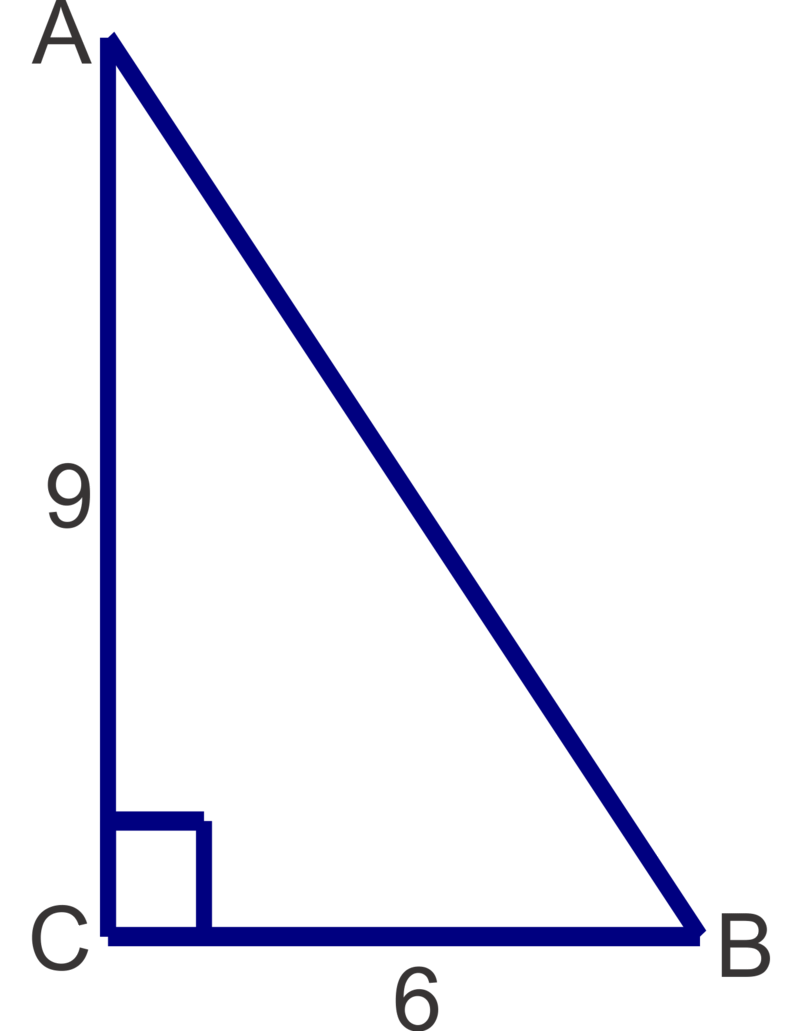Skip to main content

# 2.4.1: Inverse Trig Functions

•• Contributed by CK12
• CK12

Solving for an angle given a trigonometric ratio.

## Inverse Trig Functions and Solving Right Triangles

A right triangle has legs that measure 2 units and $$2 \sqrt{3}$$ units. What are the measures of the triangle's acute angles?

### Inverse of Trigonometric Functions

We have used the trigonometric functions sine, cosine and tangent to find the ratio of particular sides in a right triangle given an angle. In this concept we will use the inverses of these functions, $$\sin^{-1}$$, $$\cos^{-1}$$  and $$\tan^{-1}$$, to find the angle measure when the ratio of the side lengths is known. When we type $$\sin 30^{\circ}$$ into our calculator, the calculator goes to a table and finds the trig ratio associated with $$30^{\circ}$$, which is 12. When we use an inverse function we tell the calculator to look up the ratio and give us the angle measure. For example: $$\sin^{-1}\left(\dfrac{1}{2}\right)=30^{\circ}$$. On your calculator you would press $$2^{ND} SIN$$ to get $$\sin^{-1}($$ and then type in $$\dfrac{1}{2}$$, close the parenthesis and press ENTER. Your calculator screen should read $$\sin^{-1}\left(\dfrac{1}{2}\right)$$ when you press ENTER.

Let's find the measure of angle $$A$$ associated with the following ratios and round answers to the nearest degree.

1. $$\sin A=0.8336$$
2. $$\tan A=1.3527$$
3. $$\cos A=0.2785$$

Using the calculator we get the following:

1. $$\sin^{-1}(0.8336)\approx 56^{\circ}$$
2. $$\tan^{-1} (1.3527)\approx 54^{\circ}$$
3. $$\cos^{-1} (0.2785)\approx 74^{\circ}$$

Now, let's find the measures of the unknown angles in the triangle shown and round answers to the nearest degree.Figure $$\PageIndex{1}$$

We can solve for either $$x$$ or $$y$$ first. If we choose to solve for $$x$$ first, the 23 is opposite and 31 is adjacent so we will use the tangent ratio.

$$x=\tan^{-1} (\dfrac{23}{31})\approx 37^{\circ}$$.

Recall that in a right triangle, the acute angles are always complementary, so $$90^{\circ} −37^{\circ} =53^{\circ}$$, so $$y=53^{\circ}$$. We can also use the side lengths an a trig ratio to solve for y:

$$y=\tan^{-1} (\dfrac{31}{23)\approx 53^{\circ}$$.

Finally, let's solve the right triangle shown below and round all answers to the nearest tenth.Figure $$\PageIndex{2}$$

We can solve for either angle $$A$$ or angle $$B$$ first. If we choose to solve for angle B first, then 8 is the hypotenuse and 5 is the opposite side length so we will use the sine ratio.

\begin{aligned}\sin B&=\dfrac{5}{8} \\ m\angle B&=\sin^{-1}(\dfrac{5}{8})\approx 38.7^{\circ}\end{aligned}

Now we can find A two different ways.

Method 1: We can using trigonometry and the cosine ratio:

\begin{aligned}\cos A&=\dfrac{5}{8} \\ m\angle A&=\cos^{-1} (\dfrac{5}{8})\approx 51.3^{\circ}\end{aligned}

Method 2: We can subtract $$m\angle B$$ from $$90^{\circ}$$ : $$90^{\circ} −38.7^{\circ} =51.3^{\circ}$$  since the acute angles in a right triangle are always complimentary.

Either method is valid, but be careful with Method 2 because a miscalculation of angle B would make the measure you get for angle $$A$$ incorrect as well.

Example $$\PageIndex{1}$$

Earlier, you were asked to find the measures of the triangle's acute angles.

Solution

First, let's find the hypotenuse, then we can solve for either angle.

\begin{aligned} 2^2+(2\sqrt{3})&=c^2 \\ 4+12&=c^2 \\ 16&=c^2 \\ c&=4\end{aligned}

One of the acute angles will have a sine of $$\dfrac{2}{4}=\dfrac{1}{2}$$.

\begin{aligned} \sin A&=\dfrac{1}{2}\\ m\angle A&=\sin^{-1} \dfrac{1}{2}=30^{\circ}\end{aligned}

Now we can find B by subtracting $$m\angle A$$ from $$90^{\circ}$$: $$90^{\circ} −30^{\circ} =60^{\circ}$$ since the acute angles in a right triangle are always complimentary.

Example $$\PageIndex{2}$$

Find the measure of angle $$A$$.

Solution

\begin{aligned} \sin A&=0.2894 \\ \sin^{-1}(0.2894) &\approx 17^{\circ} \end{aligned}

\)Example $$\PageIndex{3}$$

Find the measure of angle $$A$$.

Solution

\begin{aligned} \tan A=2.1432 \\ \tan^{-1} (2.1432)\approx 65^{\circ} \end{aligned}

Example $$\PageIndex{4}$$

Find the measure of angle $$A$$.

Solution

\begin{aligned} \cos A&=0.8911 \\ \cos^{-1} (0.8911) &\approx 27^{\circ}\end{aligned}

Example $$\PageIndex{5}$$

Find the measures of the unknown angles in the triangle shown. Round answers to the nearest degree.Figure $$\PageIndex{3}$$

Solution

$$x=\cos^{-1} (\dfrac{13}{20})\approx 49^{\circ} ; \quad y=\sin^{-1}(\dfrac{13}{20})\approx 41^{\circ}$$

Example $$\PageIndex{6}$$

Solve the triangle. Round side lengths to the nearest tenth and angles to the nearest degree.Figure $$\PageIndex{4}$$

Solution

$$m\angle A=\cos^{-1} (\dfrac{17}{38})\approx 63^{\circ} ; m\angle B=\sin^{-1}(\dfrac{17}{38})\approx 27^{\circ} ;a=\sqrt{38^2−17^2} \approx 34.0$$

### Review

Use your calculator to find the measure of $$\angle B$$. Round answers to the nearest degree.

1. $$\tan B=0.9523$$
2. $$\sin B=0.8659$$
3. $$\cos B=0.1568$$
4. $$\sin B=0.2234$$
5. $$\cos B=0.4855$$
6. $$\tan B=0.3649$$

Find the measures of the unknown acute angles. Round measures to the nearest degree.

1.Figure $$\PageIndex{5}$$
2.Figure $$\PageIndex{6}$$
3.Figure $$\PageIndex{7}$$
4.Figure $$\PageIndex{8}$$
5.Figure $$\PageIndex{9}$$
6.Figure $$\PageIndex{10}$$

Solve the following right triangles. Round angle measures to the nearest degree and side lengths to the nearest tenth.

1.Figure $$\PageIndex{11}$$
2.Figure $$\PageIndex{12}$$
3.Figure $$\PageIndex{13}$$

### Answers for Review Problems

To see the Review answers, open this PDF file and look for section 13.3.

## Additional Resources

Interactive Element

Practice: Inverse Trig Functions

• Was this article helpful?